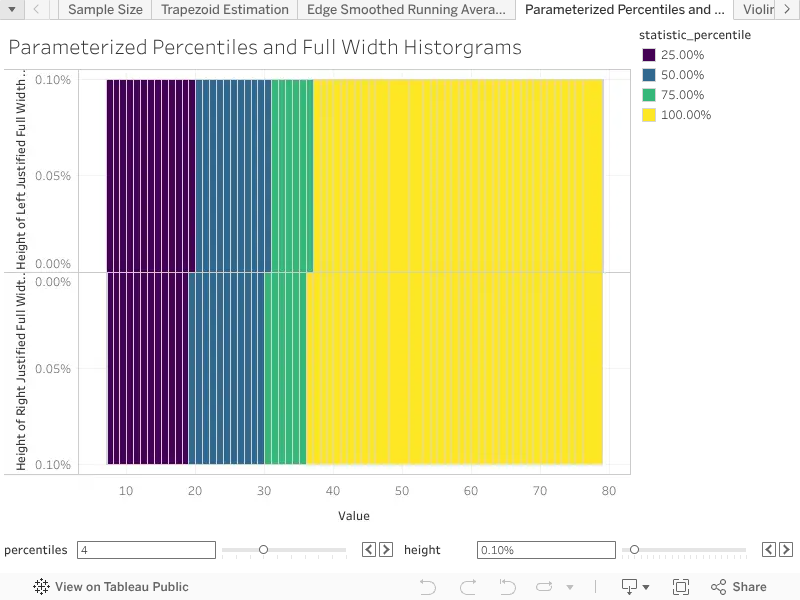# Dynamic Percentiles from Record Counts

Version 9

Description:

Tableau's WINDOW_PERCENTILE operates only on the rows, and does not take into account the implicit weighting of the rows due to the underlying record counts. Through a careful application of integer conversion we can create dynamic percentiles from record counts, using a positive parameter, typically 1 to 10. In this calculation we will classify each row by the upper bound of the percentile it belongs to, according to the running sum of the record counts in each row.

We will need to replicate the CEILING function using integer truncation, because neither FLOOR nor CEILING can accept table calculations as input.

Example Calculation:

The INT function creates left closed intervals [a, b), but we need right closed interval (a, b]. To map from left closed to right closed intervals we take modulus with respect to the number of records to test for a boundary, and then reverse the inclusion of the boundary point.

// Compute the individual percentiles from the choice of the number of percentiles.

// Integer conversion needs to be used because ceiling and floor cannot evaluate on

// table expressions.

[PERCENTILE] := IIF(

([PERCENTILES] * RUNNING_SUM(SUM(Number of Records))) % TOTAL(SUM([Number of Records])) = 0,

RUNNING_SUM(SUM(Number of Records))) / TOTAL(SUM([Number of Records])),

INT(1 + [PERCENTILES] * RUNNING_SUM(SUM(Number of Records)) / TOTAL(SUM([Number of Records]))) / [PERCENTILES]

)

Inputs and Setup:

The dynamic percentile calculation requires a parameter, in this example called [PERCENTILES], typically between one and ten.

The dynamic percentile calculation requires an addressing field to specify the order of summation.

Remember to format this field as a percentage.

Related Functions:

INDEX, SIZE, IIF, TOTAL, RUNNING_SUM, ROUND